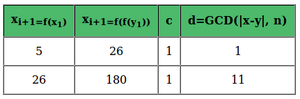Related Articles

# Java Program to Implement Pollard Rho Algorithm

• Difficulty Level : Expert
• Last Updated : 04 Jan, 2021

Pollard’s rho algorithm is an algorithm for integer factorization. It is particularly effective at splitting composite numbers with small factors. The Rho algorithm’s most remarkable success was the factorization of eighth Fermat number: 1238926361552897 * 93461639715357977769163558199606896584051237541638188580280321.  This algorithm was a good choice for F8 because the prime factor p = 1238926361552897 is much smaller than the other factor.

Example:

Attention reader! Don’t stop learning now. Get hold of all the important Java Foundation and Collections concepts with the Fundamentals of Java and Java Collections Course at a student-friendly price and become industry ready. To complete your preparation from learning a language to DS Algo and many more,  please refer Complete Interview Preparation Course.

```Input: n = 315
Output: 3 [OR 3 OR 5 OR 7]

Input: n = 10
Output: 2 [OR 5 ]```

Approach:

1. The algorithm takes as its inputs n.
2. The integer N to be factored, and g(x).
3. A polynomial in x computed modulo n.
g(x) = (x^2 + 1) % n
The output is either a non-trivial factor of n or failure.

Example:  Let us suppose n = 187, y = x = 2 and c = 1, Hence, our g(x) = x^2 + 1.11 is a non-trivial factor of 187.

Below is a Java program  to Implement Pollard Rho Algorithm:

## Java

 `// Java Program to implement Pollard’s Rho Algorithm``import` `java.io.*;`` ` `class` `GFG {`` ` `    ``int` `n = ``315``;``    ``// function to return gcd of a and b``    ``public` `int` `gcd(``int` `a, ``int` `b)``    ``{`` ` `        ``// initialise gcd = 0``        ``int` `gcd = ``0``;``        ``for` `(``int` `i = ``1``; i <= a || i <= b; i++) {``            ``if` `(a % i == ``0` `&& b % i == ``0``) {``                ``gcd = i;``            ``}``        ``}``        ``return` `gcd;``    ``}`` ` `    ``/* Function to calculate (base^exponent)%modulus */``    ``int` `g(``int` `x, ``int` `n) { ``return` `((x * x) - ``1``) % n; }`` ` `    ``public` `static` `void` `main(String args[])``    ``{`` ` `        ``GFG gfg = ``new` `GFG();`` ` `        ``int` `n = ``315``;``        ``int` `x = ``2``, y = ``2``, d = ``1``;`` ` `        ``while` `(d == ``1``) {`` ` `            ``// Tortoise Move``            ``x = gfg.g(x, n);`` ` `            ``// Hare Move:``            ``y = gfg.g(gfg.g(y, n), n);`` ` `            ``/* check gcd of |x-y| and n */``            ``d = gfg.gcd((x - y), gfg.n);``        ``}`` ` `        ``// if the algorithm fails to find prime factor``        ``if` `(d == gfg.n) {``            ``System.out.println(``                ``"GCD cannot be found for this element"``);``        ``}``        ``else` `{``            ``System.out.println(``"One of the prime factor of "``                               ``+ n + ``" is "` `+ d);``        ``}``    ``}``}`
Output
```One of the prime factor of 315 is 5
```

Time Complexity: O(sqrt(n))

My Personal Notes arrow_drop_up